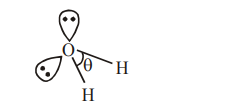# Given below are two statements : one is labelled as Assertion A and the other is labelled as Reason R :Question:

Given below are two statements : one is labelled as Assertion A and the other is labelled as Reason R :

Assertion A : The H-O-H bond angle in water molecule is $104.5^{\circ}$.

Reason $\mathbf{R}$ : The lone pair - lone pair repulsion of electrons is higher than the bond pair - bond pair repulsion.

1. A is false but R is true

2.  Both A and R are true, but R is not the correct correct explanation of A

3. A is true but R is false

4. Both A and R are true, and R is the correct explanation of A

Correct Option: 4,

Solution:

$\mathrm{H}_{2} \mathrm{O}$$\theta=104.5^{\circ}$

the hybridisation of oxygen is water molecule is $\mathrm{sp}^{3}$.

So electron geometry of water molecule is tetrahedral and the bond angle should be 109°28" but as we know that lone pair-lone pair repulsion of electrons is higher than the bond pair-bond pair repulsion because lone pair is occupied more space areound central atom than that of bond pair.